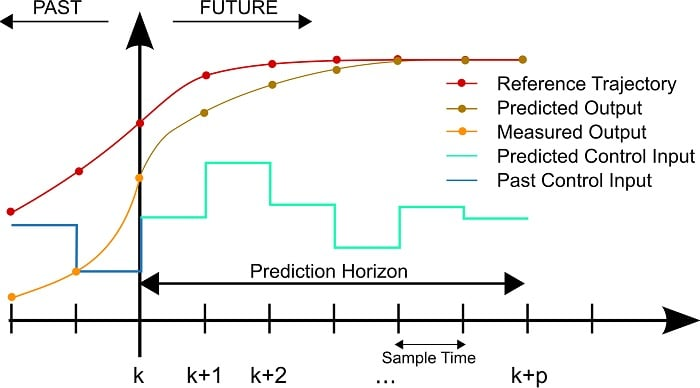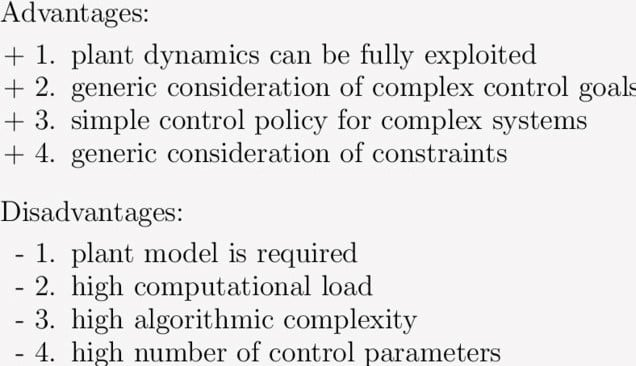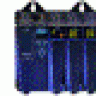Technical Article

# What is Model Predictive Control (MPC)?

August 10, 2020 by Mitesh Agrawal

## This article discusses the applications and uses of model predictive control.

Over the years, control systems for robotics systems have drastically changed to allow for more complex actions and nonlinear dynamics. Model predictive control is one strategy to allow for these more complex behaviors.

All these applications involve either dynamic environments or dangerous inaccessible environments that do not allow for human intervention. To add, most of these robot models are highly nonlinear making control strategies more difficult.

Typical industrial control strategies such as PD control and PID control can fail in guaranteeing many kinds of features. Though there is a lot of research with different optimal control strategies for requirements such as adaptive control and imitation control, one control strategy clearly stands out in the state of the art research in the domain.

It is Model Predictive Control (MPC), which has taken years of researchers developing control strategies curated specifically for different applications. This article will establish the basic fundamentals before picking up MPC.

### What Is MPC?

Imagine walking in a dark room. You try to sense the surroundings, predict the best path in the direction of a goal, but take only one step at a time and repeat the cycle.

Similarly, the MPC process is like walking into a dark room. The essence of MPC is to optimize the manipulatable inputs and the forecasts of process behavior.

MPC is an iterative process of optimizing the predictions of robot states in the future limited horizon while manipulating inputs for a given horizon. The forecasting is achieved using the process model. Thus, a dynamic model is essential while implementing MPC. These process models are generally nonlinear, but for short periods of time, there are methods such as tailor expansion to linearize these models.

These approximations in linearization or unpredictable changes in dynamic models might cause errors in forecasting. Thus, MPC only takes action on first computed control input and then recalculates the optimized forecasts based on feedback. This implies MPC is an iterative, model-based, predictive, optimal, and feedback based control strategy.

### How Does MPC Work?

MPC has three basic requirements to work. The first one is a cost function J, which describes the expected behavior of the robot.

This generally involves parameters of comparison between different possibilities of actions, such as minimization of error from the reference trajectory, minimization of jerk, obstacle avoidance, etc.

##### In general, cost function can be visualized as shown above.

Where

J: cost function

xt: robot states at time t

rt: robot reference states at time t

ut: robot predicted input at time t

Wt and Wb: Weights according to the requirement

The above cost function minimizes error from a reference trajectory as well as jerk caused by drastic deviations in inputs to the robot.

The second requirement is a dynamic model of the robot. This dynamic model enables MPC to simulate states of a robot in a given horizon with different possibilities of inputs. The third is the optimization algorithm used to solve given optimization function J. Along with these requirements, MPC provides flexibility to mention certain constraints to be taken into consideration while performing optimization. These constraints can be the minimum and maximum value of states and inputs to the robot.##### A basic working principle of MPC. Image courtesy of Martin Behrendt.

In order to understand the working of MPC consider the robot is at current time k in the simulated robot movement and has a reference trajectory that needs to be followed for a given horizon p.

MPC takes current states of the robot as input and simulates possibilities of control inputs for time k to k+p. From different possibilities, MPC selects the best series of inputs that minimize the cost function. From this series of predicted control input, MPC then implements only the first input and repeats the cycle at time k+1. Due to these iterative cycles over the horizon taking one step at a time, MPC is also called receding horizon control. This receding control can be better observed in the given simulation where black markers represent desired trajectories and red markers represent forecasted trajectories from MPC.

MPC has the biggest advantage of exploiting plant dynamics as it explores all or most available options of control input depending upon optimization algorithms. The second biggest advantage is its flexibility in achieving complex goals and implementing robust robot constraints. Depending on the requirement, there is a lot of room to curate task-specific objective function and apply design limit specific constraints on inputs, as well as predicted outputs of a robot. MPC, thus gives a very simple control strategy for complex control systems.

Along with its advantages, MPC has a list of disadvantages too. Depending on optimization algorithms and dynamic models of robots, MPC has a huge drawback of computational complexity because of iterative calculations at each time step. There are a lot of methods to reduce these computational complexities such as the following:

1. Warm start i.e. using previous calculation as a base for optimization at the next step.
2. Primal log barrier which reduces inequality constraints as a part of the objective function.
3. Use of efficient optimization methods such as Newton’s method.##### A diagram showing some of the advantages and disadvantages of MPC.

Secondly, a dynamic model of the robot is required for MPC which may not be very easy to derive for complex robots. Also, MPC has a drawback of having a high number of control variables.

### Applications of MPC

MPC has been efficiently used in several industrial processes, such as chemical plants or oil refineries. However, its application to robotic systems in a true industrial environment, in which unavoidable modeling uncertainties and external disturbances affect the system, is still limited.

ABB is using MPC to help clients from different sectors such as mining, minerals, cement, pulp and paper, oil and gas, and marine sectors. In the world of robotics, MPC is most commonly used for the planning and control of autonomous vehicles. Robots with high levels of autonomy and nonlinearities in dynamic models such as space robots and airplanes use MPC.

Another example of such a complex robot is a mobile manipulator. These robots must maintain their stability in dynamic environments that call for the use of MPC. Various warehouse management manipulators with dynamic loading use MPC because of instability issues while using PID or adaptive control strategies.

MPC is also used in plants with precise machine tooling operations where there are no human interventions. In such cases, either the material of cutting tools is very costly and without human interventions, errors might lead to huge losses. Such precise movements are achieved better using MPC. Robot manipulators with an eye in hand are becoming more popular applications in these industries. As there is a high level of uncertainty associated with these robots, MPC is one of the most commonly used control strategies.•pnachtwey August 13, 2020
•Water November 27, 2022